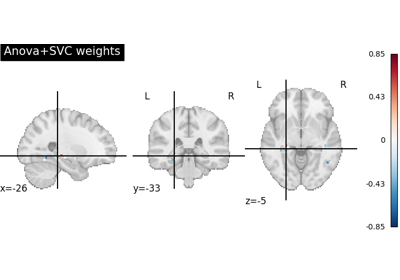Note

This page is a reference documentation. It only explains the class signature, and not how to use it. Please refer to the user guide for the big picture.

# nilearn.decoding.Decoder#

class nilearn.decoding.Decoder(estimator='svc', mask=None, cv=10, param_grid=None, screening_percentile=20, scoring='roc_auc', smoothing_fwhm=None, standardize=True, target_affine=None, target_shape=None, mask_strategy='background', low_pass=None, high_pass=None, t_r=None, memory=None, memory_level=0, n_jobs=1, verbose=0)[source]#

A wrapper for popular classification strategies in neuroimaging.

The Decoder object supports classification methods. It implements a model selection scheme that averages the best models within a cross validation loop. The resulting average model is the one used as a classifier. This object also leverages the`NiftiMaskers` to provide a direct interface with the Nifti files on disk.

Parameters
estimator: str, optional

The estimator to choose among:

Default ‘svc’.

Mask to be used on data. If an instance of masker is passed, then its mask and parameters will be used. If no mask is given, mask will be computed automatically from provided images by an inbuilt masker with default parameters. Refer to NiftiMasker or MultiNiftiMasker to check for default parameters. Default None

cv: cross-validation generator or int, optional (default 10)

A cross-validation generator. See: https://scikit-learn.org/stable/modules/cross_validation.html

param_grid: dict of str to sequence, or sequence of such. Default None

The parameter grid to explore, as a dictionary mapping estimator parameters to sequences of allowed values.

None or an empty dict signifies default parameters.

A sequence of dicts signifies a sequence of grids to search, and is useful to avoid exploring parameter combinations that make no sense or have no effect. See scikit-learn documentation for more information, for example: https://scikit-learn.org/stable/modules/grid_search.html

For DummyClassifier, parameter grid defaults to empty dictionary, class predictions are estimated using default strategy.

screening_percentile: int, float, optional, in the closed interval [0, 100]

The percentage of brain volume that will be kept with respect to a full MNI template. In particular, if it is lower than 100, a univariate feature selection based on the Anova F-value for the input data will be performed. A float according to a percentile of the highest scores. Default: 20.

scoring: str, callable or None, optional. Default: ‘roc_auc’

The scoring strategy to use. See the scikit-learn documentation at https://scikit-learn.org/stable/modules/model_evaluation.html#the-scoring-parameter-defining-model-evaluation-rules If callable, takes as arguments the fitted estimator, the test data (X_test) and the test target (y_test) if y is not None. e.g. scorer(estimator, X_test, y_test)

For classification, valid entries are: ‘accuracy’, ‘f1’, ‘precision’, ‘recall’ or ‘roc_auc’. Default: ‘roc_auc’.

smoothing_fwhm`float`, optional.

If `smoothing_fwhm` is not `None`, it gives the full-width at half maximum in millimeters of the spatial smoothing to apply to the signal.

standardize`bool`, optional.

If `standardize` is True, the data are centered and normed: their mean is put to 0 and their variance is put to 1 in the time dimension. Default=True.

target_affine`numpy.ndarray`, optional.

If specified, the image is resampled corresponding to this new affine. `target_affine` can be a 3x3 or a 4x4 matrix. Default=None.

target_shape`tuple` or `list`, optional.

If specified, the image will be resized to match this new shape. `len(target_shape)` must be equal to 3.

Note

If `target_shape` is specified, a `target_affine` of shape `(4, 4)` must also be given.

Default=None.

low_pass`float` or None, optional

Low cutoff frequency in Hertz. If specified, signals above this frequency will be filtered out. If None, no low-pass filtering will be performed. Default=None.

high_pass`float`, optional

High cutoff frequency in Hertz. If specified, signals below this frequency will be filtered out. Default=None.

t_r`float` or None, optional

Repetition time, in seconds (sampling period). Set to `None` if not provided. Default=None.

The strategy used to compute the mask:

• ‘background’: Use this option if your images present a clear homogeneous background.

• ‘epi’: Use this option if your images are raw EPI images

• ‘whole-brain-template’: This will extract the whole-brain part of your data by resampling the MNI152 brain mask for your data’s field of view.

Note

This option is equivalent to the previous ‘template’ option which is now deprecated.

• ‘gm-template’: This will extract the gray matter part of your data by resampling the corresponding MNI152 template for your data’s field of view.

New in version 0.8.1.

• ‘wm-template’: This will extract the white matter part of your data by resampling the corresponding MNI152 template for your data’s field of view.

New in version 0.8.1.

Note

This parameter will be ignored if a mask image is provided.

Default is ‘background’.

memoryinstance of `joblib.Memory`, `str`, or `pathlib.Path`

Used to cache the masking process. By default, no caching is done. If a `str` is given, it is the path to the caching directory.

memory_level`int`, optional.

Rough estimator of the amount of memory used by caching. Higher value means more memory for caching. Zero means no caching. Default=0.

n_jobs`int`, optional.

The number of CPUs to use to do the computation. -1 means ‘all CPUs’. Default=1.

verbose`int`, optional

Verbosity level (0 means no message). Default=0.

`nilearn.decoding.DecoderRegressor`

regression strategies for Neuro-imaging,

`nilearn.decoding.FREMClassifier`

State of the art classification pipeline for Neuroimaging

`nilearn.decoding.SpaceNetClassifier`

Graph-Net and TV-L1 priors/penalties

__init__(estimator='svc', mask=None, cv=10, param_grid=None, screening_percentile=20, scoring='roc_auc', smoothing_fwhm=None, standardize=True, target_affine=None, target_shape=None, mask_strategy='background', low_pass=None, high_pass=None, t_r=None, memory=None, memory_level=0, n_jobs=1, verbose=0)[source]#
decision_function(X)[source]#

Predict class labels for samples in X.

Parameters
X: list of Niimg-like objects

See Input and output: neuroimaging data representation. Data on prediction is to be made. If this is a list, the affine is considered the same for all.

Returns
y_pred: ndarray, shape (n_samples,)

Predicted class label per sample.

fit(X, y, groups=None)[source]#

Fit the decoder (learner).

Parameters
X: list of Niimg-like objects

See Input and output: neuroimaging data representation. Data on which model is to be fitted. If this is a list, the affine is considered the same for all.

y: numpy.ndarray of shape=(n_samples) or list of length n_samples

The dependent variable (age, sex, IQ, yes/no, etc.). Target variable to predict. Must have exactly as many elements as 3D images in niimg.

groups: None

Group labels for the samples used while splitting the dataset into train/test set. Default None.

Note that this parameter must be specified in some scikit-learn cross-validation generators to calculate the number of splits, e.g. sklearn.model_selection.LeaveOneGroupOut or sklearn.model_selection.LeavePGroupsOut.

Attributes

`classes_`numpy.ndarray

Classes to predict. For classification only.

`screening_percentile_`float

Screening percentile corrected according to volume of mask, relative to the volume of standard brain.

`coef_`numpy.ndarray, shape=(n_classes, n_features)

Contains the mean of the models weight vector across fold for each class. Returns None for Dummy estimators.

`coef_img_`dict of Nifti1Image

Dictionary containing coef_ with class names as keys, and coef_ transformed in Nifti1Images as values. In the case of a regression, it contains a single Nifti1Image at the key ‘beta’. Ignored if Dummy estimators are provided.

`intercept_`ndarray, shape (nclasses,)

Intercept (a.k.a. bias) added to the decision function. Ignored if Dummy estimators are provided.

`cv_`list of pairs of lists

List of the (n_folds,) folds. For the corresponding fold, each pair is composed of two lists of indices, one for the train samples and one for the test samples.

`std_coef_`numpy.ndarray, shape=(n_classes, n_features)

Contains the standard deviation of the models weight vector across fold for each class. Note that folds are not independent, see https://scikit-learn.org/stable/modules/cross_validation.html#cross-validation-iterators-for-grouped-data Ignored if Dummy estimators are provided.

`std_coef_img_`dict of Nifti1Image

Dictionary containing std_coef_ with class names as keys, and coef_ transformed in Nifti1Image as values. In the case of a regression, it contains a single Nifti1Image at the key ‘beta’. Ignored if Dummy estimators are provided.

`cv_params_`dict of lists

Best point in the parameter grid for each tested fold in the inner cross validation loop. The grid is empty when Dummy estimators are provided.

‘scorer_’function

Scorer function used on the held out data to choose the best parameters for the model.

`cv_scores_`dict, (classes, n_folds)

Scores (misclassification) for each parameter, and on each fold

`n_outputs_`int

Number of outputs (column-wise)

`dummy_output_`: ndarray, shape=(n_classes, 2) or shape=(1, 1) for regression

Contains dummy estimator attributes after class predictions using strategies of DummyClassifier (class_prior) and DummyRegressor (constant) from scikit-learn. This attribute is necessary for estimating class predictions after fit. Returns None if non-dummy estimators are provided.

get_params(deep=True)#

Get parameters for this estimator.

Parameters
deepbool, default=True

If True, will return the parameters for this estimator and contained subobjects that are estimators.

Returns
paramsdict

Parameter names mapped to their values.

predict(X)[source]#

Predict a label for all X vectors indexed by the first axis.

Parameters
X: {array-like, sparse matrix}, shape = (n_samples, n_features)

Samples.

Returns
array, shape=(n_samples,) if n_classes == 2 else (n_samples, n_classes)

Confidence scores per (sample, class) combination. In the binary case, confidence score for self.classes_ where >0 means this class would be predicted.

score(X, y, *args)[source]#

Compute the prediction score using the scoring metric defined by the scoring attribute.

Parameters
X{array-like, sparse matrix}, shape = (n_samples, n_features)

Samples.

yarray-like

Target values.

argsOptional arguments that can be passed to

scoring metrics. Example: sample_weight.

Returns
scorefloat

Prediction score.

set_params(**params)#

Set the parameters of this estimator.

The method works on simple estimators as well as on nested objects (such as `Pipeline`). The latter have parameters of the form `<component>__<parameter>` so that it’s possible to update each component of a nested object.

Parameters
**paramsdict

Estimator parameters.

Returns
selfestimator instance

Estimator instance.

## Examples using `nilearn.decoding.Decoder`#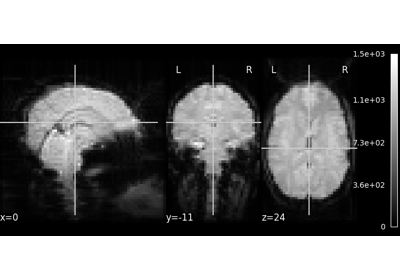A introduction tutorial to fMRI decoding

A introduction tutorial to fMRI decoding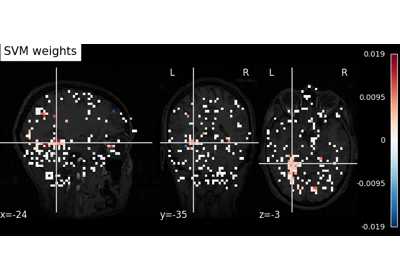Decoding with ANOVA + SVM: face vs house in the Haxby dataset

Decoding with ANOVA + SVM: face vs house in the Haxby dataset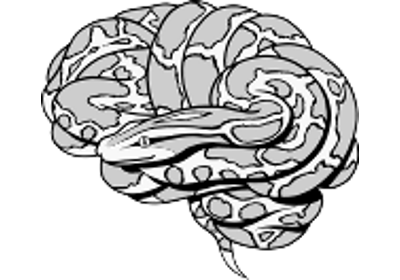Decoding of a dataset after GLM fit for signal extraction

Decoding of a dataset after GLM fit for signal extraction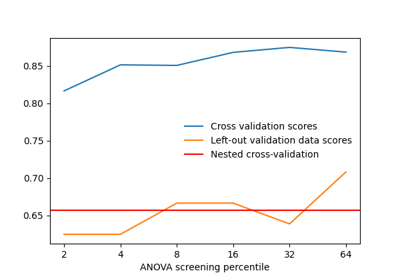Setting a parameter by cross-validation

Setting a parameter by cross-validation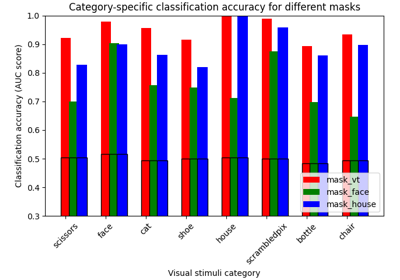ROI-based decoding analysis in Haxby et al. dataset

ROI-based decoding analysis in Haxby et al. dataset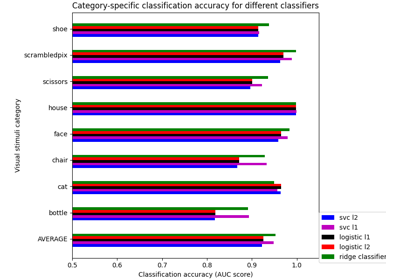Different classifiers in decoding the Haxby dataset

Different classifiers in decoding the Haxby dataset